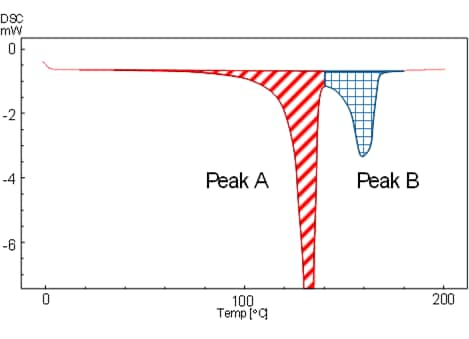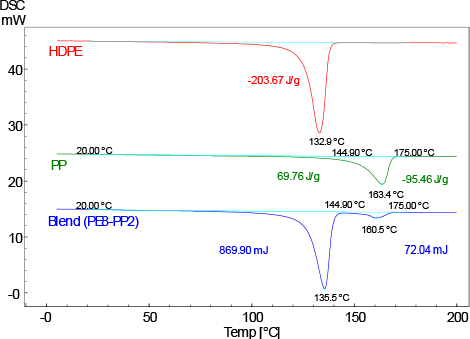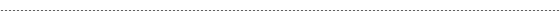# Measurement of Polyethylene (PE)-Polypropylene (PP) Blend Samples

Mechanically blended polymeric materials exhibit the characteristics of each component, such as melting and crystallization, and multiple changes derived from each component can be observed through measurement with a DSC (differential scanning calorimeter).
This research utilizes this process to introduce examples of determining the component ratios of blended high molecular materials by measuring the heat of fusion with a DSC.Fig. 1 Obtaining the Approximate Heat of FusionFig. 2 DSC Curves of HDPE, PP, and Blend Sample

Since the melting peaks of HDPE and PP are detected at very close temperatures, the peaks overlap and cannot be completely separated. In this case, the peaks are divided laterally as shown in Fig. 1 and the heat quantity (approximated) of each peak is calculated as the heat of fusion of each component. (An optional partial area analysis program is required to perform this calculation.)### Differential Scanning Calorimeter（DSC-60 Plus Series）

The temperature difference between a standard substance and a test sample is measured while applying constant heat. The endothermic reactions and exothermic reactions are measured, which can be used for physical evaluation of polymer materials, metals, etc. When microplastics are measured, the types can be identified and component percentages obtained.